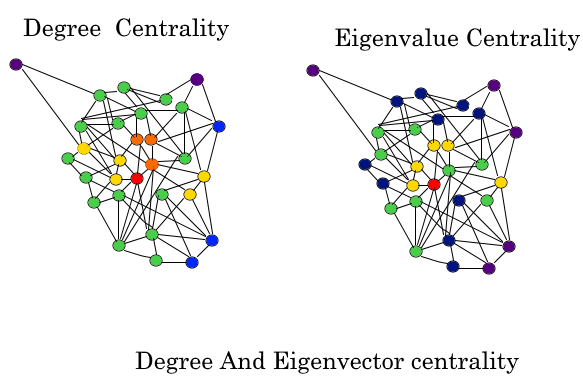Related Articles
Eigenvector Centrality (Centrality Measure)
• Difficulty Level : Medium
• Last Updated : 12 Feb, 2018

In graph theory, eigenvector centrality (also called eigencentrality) is a measure of the influence of a node in a network. It assigns relative scores to all nodes in the network based on the concept that connections to high-scoring nodes contribute more to the score of the node in question than equal connections to low-scoring nodes.

Google’s PageRank and the Katz centrality are variants of the eigenvector centrality.

Using the adjacency matrix to find eigenvector centrality

For a given graphwithvertices letbe the adjacency matrix, i.e.if vertexis linked to vertex, andotherwise. The relative centrality score of vertexcan be defined as:whereis a set of the neighbors ofandis a constant. With a small rearrangement this can be rewritten in vector notation as the eigenvector equationIn general, there will be many different eigenvaluesfor which a non-zero eigenvector solution exists. However, the additional requirement that all the entries in the eigenvector be non-negative implies (by the Perron–Frobenius theorem) that only the greatest eigenvalue results in the desired centrality measure. Thecomponent of the related eigenvector then gives the relative centrality score of the vertexin the network. The eigenvector is only defined up to a common factor, so only the ratios of the centralities of the vertices are well defined. To define an absolute score one must normalise the eigen vector e.g. such that the sum over all vertices is 1 or the total number of vertices n. Power iteration is one of many eigenvalue algorithms that may be used to find this dominant eigenvector. Furthermore, this can be generalized so that the entries in A can be real numbers representing connection strengths, as in a stochastic matrix.Following is the code for the calculation of the Eigen Vector Centrality of the graph and its various nodes.

 def eigenvector_centrality(G, max_iter=100, tol=1.0e-6, nstart=None,                           weight='weight'):    """Compute the eigenvector centrality for the graph G.      Eigenvector centrality computes the centrality for a node based on the    centrality of its neighbors. The eigenvector centrality for node i is      .. math::          \mathbf{Ax} = \lambda \mathbf{x}      where A is the adjacency matrix of the graph G with eigenvalue \lambda.    By virtue of the Perron–Frobenius theorem, there is a unique and positive    solution if \lambda is the largest eigenvalue associated with the    eigenvector of the adjacency matrix A (_).      Parameters    ----------    G : graph      A networkx graph      max_iter : integer, optional      Maximum number of iterations in power method.      tol : float, optional      Error tolerance used to check convergence in power method iteration.      nstart : dictionary, optional      Starting value of eigenvector iteration for each node.      weight : None or string, optional      If None, all edge weights are considered equal.      Otherwise holds the name of the edge attribute used as weight.      Returns    -------    nodes : dictionary       Dictionary of nodes with eigenvector centrality as the value.              Notes    ------    The eigenvector calculation is done by the power iteration method and has    no guarantee of convergence. The iteration will stop after max_iter    iterations or an error tolerance of number_of_nodes(G)*tol has been    reached.      For directed graphs this is "left" eigenvector centrality which corresponds    to the in-edges in the graph. For out-edges eigenvector centrality    first reverse the graph with G.reverse().           """    from math import sqrt    if type(G) == nx.MultiGraph or type(G) == nx.MultiDiGraph:        raise nx.NetworkXException("Not defined for multigraphs.")      if len(G) == 0:        raise nx.NetworkXException("Empty graph.")      if nstart is None:          # choose starting vector with entries of 1/len(G)        x = dict([(n,1.0/len(G)) for n in G])    else:        x = nstart      # normalize starting vector    s = 1.0/sum(x.values())    for k in x:        x[k] *= s    nnodes = G.number_of_nodes()      # make up to max_iter iterations    for i in range(max_iter):        xlast = x        x = dict.fromkeys(xlast, 0)          # do the multiplication y^T = x^T A        for n in x:            for nbr in G[n]:                x[nbr] += xlast[n] * G[n][nbr].get(weight, 1)          # normalize vector        try:            s = 1.0/sqrt(sum(v**2 for v in x.values()))          # this should never be zero?        except ZeroDivisionError:            s = 1.0        for n in x:            x[n] *= s          # check convergence        err = sum([abs(x[n]-xlast[n]) for n in x])        if err < nnodes*tol:            return x      raise nx.NetworkXError("""eigenvector_centrality():power iteration failed to converge in %d iterations."%(i+1))""")

The above function is invoked using the networkx library and once the library is installed, you can eventually use it and the following code has to be written in python for the implementation of the eigen vector centrality of a node.

 >>> import networkx as nx>>> G = nx.path_graph(4)>>> centrality = nx.eigenvector_centrality(G)>>> print(['%s %0.2f'%(node,centrality[node]) for node in centrality])

The output of the above code is:

 ['0 0.37', '1 0.60', '2 0.60', '3 0.37']

The above result is a dictionary depicting the value of eigen vector centrality of each node. The above is an extension of my article series on the centrality measures. Keep networking!!!

References
You can read more about the same at

https://en.wikipedia.org/wiki/Eigenvector_centrality

http://networkx.readthedocs.io/en/networkx-1.10/index.html

Attention reader! Don’t stop learning now. Get hold of all the important DSA concepts with the DSA Self Paced Course at a student-friendly price and become industry ready.

My Personal Notes arrow_drop_up